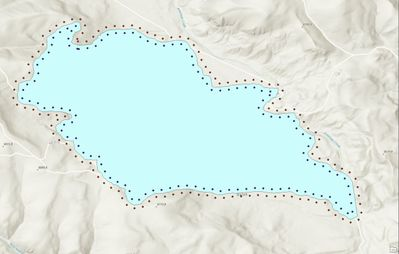# Pairing Points in Two Datasets

329
3
10-14-2022 10:16 AMOccasional Contributor II

Hello

I have two datasets of points that exist on either side of a buffered line (one that resides inside of a buffer and one that resides outside). I am going to take values of each point based on vegetation classes found in an underlying raster layer and compare those that are directly across from one another along the buffered line.

What I am trying to work out is how I can match the points that are across from one another into a new dataset?  Or, is there a way I can create a common field with a common value that will can be shared between the adjacent points that will ensure that the points that are directly across from one another will inherit that same value?

Here is a pic of the points that I am trying to match for further analysis:Thanks

3 RepliesbyMVP Esteemed Contributor

Depends on how the points were generated and if the cross-line distance is shorter than the interpoint distance. In that case

Near (Analysis)—ArcGIS Pro | Documentation

may work, fully, or at least provide you with most of the pairing ID values to enable generating the pairs from there.

since it provides the id and x, y values for the closest point

... sort of retired...byMVP Notable Contributor

I agree with @DanPatterson , you need to explain how these points were created in the first place.  A simple visual scan of your image and it is obvious there is NOT a 1-1 match. I highlight several examples in your image. How do you want to process these?How were these points created, why have you allowed  to have points unmatched? As Dan hints a near tool will erroneously identify points because some will be nearer to their adjacent rather than cross border.

Finally how many lakes are to be processed, if it really is this one, by hand may be quicker rather than develop up a workflow to capture all cases?byMVP Frequent Contributor

Your points don't actually pair up (look at the sharp corners), so you'll have problems getting a 1-1 relationship to work.

If you have to use these points, you can use CalculateField with an Arcade expression like this:

``````// Calculate a new field on PointFC_1 that stores the OBJECTID of the closest Point in PointFC_2

var point_fc_2 = FeaturesetByName(\$datastore, "PointFC_2")

// get points close to the current point, choose a buffer value that is big enough to get at least 1 other point!
var p_buffer = Buffer(\$feature, 1000, "meters")
var close_points = Intersects(point_fc_2, p_buffer)

// loop through the points and find the ID of the closest one
var min_dist = 99999
var closest_oid = null
for(var p in close_points) {
var dist = Distance(p, \$feature)
if(dist < min_dist) {
min_dist = dist
closest_oid = p.OBJECTID
}
}
return closest_oid``````

This will leave some points in FC2 without partner and it will give some points in FC1 the same partner.

If you want to get a 1-1 relationship, you have to generate your points differently. Copy and edit this Python script, then run it in the Python window:

``````polygon_layer = "TestPolygons"
point_distance = 500
buffer_distance = 100

# create output fc
points = arcpy.management.CreateFeatureclass("", "PairedPoints", "POINT")

# generate points ON the line
points_on_lines = arcpy.management.GeneratePointsAlongLines(polygon_layer, "PointsOnLines", 'DISTANCE', point_distance)

# read the polygon geometries as dict {OBJECTID: Geometry}
polygon_shapes = {p: p for p in arcpy.da.SearchCursor(polygon_layer, ["OID@", "SHAPE@"])}

# start inserting points into the output fc
with arcpy.da.InsertCursor(points, ["SHAPE@", "PolygonOID", "PointPairID", "Type"]) as i_cursor:
# loop through the points on the line
with arcpy.da.SearchCursor(points_on_lines, ["SHAPE@", "ORIG_FID", "OID@"]) as s_cursor:
for shp, poly_id, point_id in s_cursor:
# get the polygon this point is on
poly_shp = polygon_shapes[poly_id]
# create a small buffer around the point
p_buffer = shp.buffer(0.01)
# get the 2 points where the buffer intersects with the polygon
i_points = p_buffer.intersect(poly_shp, 1)
sr = poly_shp.spatialReference
fp = arcpy.PointGeometry(i_points.firstPoint, sr)
lp = arcpy.PointGeometry(i_points.lastPoint, sr)
# calculate the angle between those points -> line angle at the curretn point
angle = fp.angleAndDistanceTo(lp)
# create two points perpendicular to the line
p1 = shp.pointFromAngleAndDistance(angle + 90, buffer_distance)
p2 = shp.pointFromAngleAndDistance(angle + 90, -buffer_distance)
# calculate if the points are inside or outside the polygon
p1_type = "OUTSIDE" if p1.disjoint(poly_shp) else "INSIDE"
p2_type = "INSIDE" if p1_type == "OUTSIDE" else "OUTSIDE"
# insert both points
i_cursor.insertRow([p1, poly_id, point_id, p1_type])
i_cursor.insertRow([p2, poly_id, point_id, p2_type])``````Have a great day!
Johannes